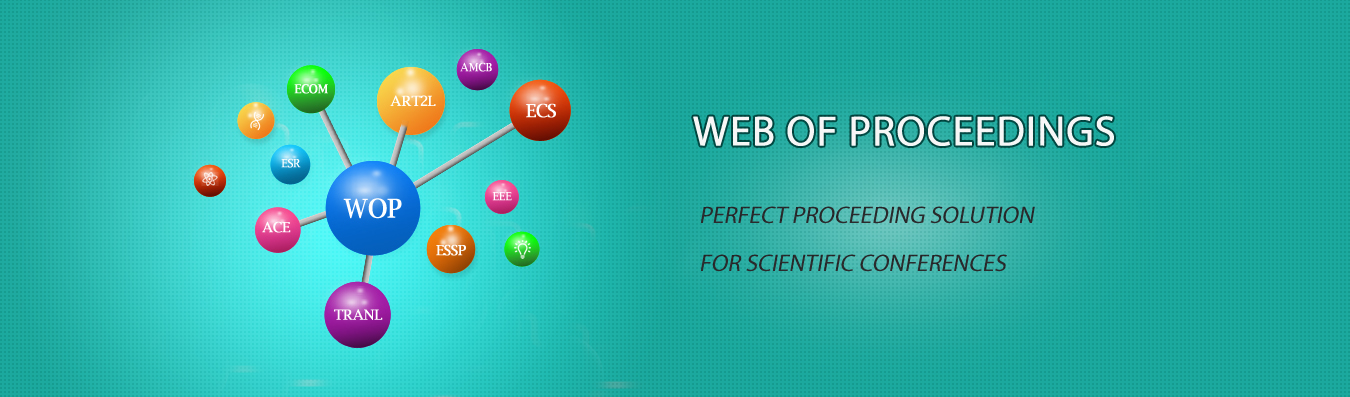The best way to conference proceedings by Francis Academic Press### Research on the Path of Integrating Mathematics Software and Mathematics Modeling into Mathematics Teaching

DOI: 10.25236/ICRTPE.2019.038

Yunpeng Huang

Yunpeng Huang

#### Abstract

In order to adapt to the development of school education, college mathematics has undergone great reform. But in the process of school education practice, there is still a big gap between the effect of mathematics teaching and the goal of actual education. This paper deeply analyses the practical value of integrating mathematical software and mathematical modeling into mathematics teaching course, combines mathematical software with university teaching content, and solves complex computational and functional mapping problems, so as to improve students’ learning enthusiasm and self-confidence. At the same time, teachers should combine mathematical software with mathematical modeling in mathematics teaching, cultivate students’ mathematical thinking quality and application ability of mathematical knowledge, and further optimize the content of mathematics teaching.

#### Keywords

Mathematical software; Mathematical modeling; Mathematical teaching; Practice; application path